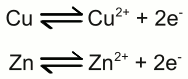# Galvanic cell

## Half cell

A metal electrode dipped into an aqueous solution of a salt of the metal is called a half cell. An example for such a half cell is a copper electrode dipped into an aqueous solution of cupric chloride. As soon as the electrode is diving into the electrolyte, the following chemical reaction occurs:Copper atoms enter the electrolyte as positively charged Cu2+ cations, each of them leaving two electrons at the electrode. As a result of the reaction, the electrode gets negatively charged:

Figure 1:
Copper in an aquatic solution of cupric chloride:
The super-numerous electrons inside of the electrode arrange in such a way, that the electric field inside of the conductive material is canceled. As a result of this ambition, they stop moving at the surface of the electrode. A layer of positively charged copper cations is attracted to the surface of the electrode by the coulomb force, preventing more copper atoms from entering the solution as ions. The state of chemical equilibrium is reached. The number of copper ions entering the solution, equals those of the copper atoms being deposited at the surface of the electrode.

The potential difference between the net positive charge of the electrolyte and the negative charge of the electrode at the state of equilibrium is primarily characterized by the used combination of metal and electrolyte. The tendency of different metals, to dissolve in an aqueous solution of it's salt, differs. The nobler a metal is, the lower is the number of atoms entering the solution.

## Galvanic cell

An arrangement of two half cells being connected by a salt bridge is called a galvanic cell. Each half cell consists of a metal and a solution of the salt of the metal:

Figure 2:
Galvanic cell:
The left half cell consists of a zinc electrode and a solution of zinc sulfate, the right half cell of a copper electrode and a solution of copper sulfate. Copper is nobler than zinc, so the number of zinc atoms entering the left half cell as positively charged ions is higher than those of the copper atoms entering the solution at the right half cell.
The chemical reactions at the half cells are given by the equations:Between both electrodes of the half cells, a voltage difference can be detected. When the two electrodes are connected by a wire, electrons move from the zinc electrode to the copper electrode, as a consequence of the voltage difference. A lower number of electrons inside of the zinc electrode leads to a lower number of zinc ions surrounding the electrode, bound by the (lower) coulomb force. As a consequence of that, more zinc atoms can enter the solution as ions. Vice versa a higher number of electrons inside of the copper electrode, leads to a higher number of cupric ions being attracted by the copper electrode, hence coper ions leave the solution and are deposited as copper atoms at the surface of the electrode. In turn, the electrolyte of the left half cell gets positively charged, the electrolyte of the right half cell gets negatively charged. That's why the salt bridge is needed to keep the current flow. Sulfate anions can move from the right half cell to the left half cell, to balance the charge difference by using the salt bridge as connection.

Figure 3:
Short circuit between two galvanic half cells:
Driven by the potential difference between the electrodes of the two half cells, electrons are moving from the zinc electrode to the copper electrode. To balance the charge difference between the half cells, twice negatively charged sulfate ions are moving from the right half cell to the left one through the salt bridge.

The chemical reaction stops either as soon as the zinc electrode is completely oxidized to zinc sulfate, or all copper ions are reduced to elemental copper atoms. The more noble metal oxidizes the less noble metal in a redox process:The voltage of the galvanic cell equals the difference of the voltages of the two half-cells, which can be determined by using the standard potential table. At the example given above, the half reaction between Cu and Cu2+ gives +0,35V and the half reaction between Zn and Zn2+ gives -0,76V. When subtracting both values from each other, we get: +0,35V - (-0,76V) = +1,11V.